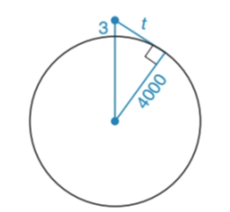Chapter 6.2, Problem 27EElementary Geometry For College St...

7th Edition
Alexander + 2 others
ISBN: 9781337614085

Solutions

Chapter
SectionElementary Geometry For College St...

7th Edition
Alexander + 2 others
ISBN: 9781337614085
Textbook Problem

An airplane reaches an altitude of 3 mi above the earth. Assuming a clear day and that a passenger has binoculars, how far can the passengers see?(HINT: The radius of the earth is approximately 4000 mi.)To determine

To find:

The distance that the passengers able to see.

Explanation

Given:

The radius of earth 4000mi.

Calculation:

The place reaches an altitude 3mi above the earth.

In the given figure we can observe the right triangle.

By Pythagorean theorem.

(4000+3)2=40002+t2(4003)2=4000</

Still sussing out bartleby?

Check out a sample textbook solution.

See a sample solution

The Solution to Your Study Problems

Bartleby provides explanations to thousands of textbook problems written by our experts, many with advanced degrees!

Get Started

Prove, using Definition 9, that limxx3=. Definition 9

Single Variable Calculus: Early Transcendentals, Volume I

In Exercises 69-74, rationalize the numerator. 69. 2x3

Applied Calculus for the Managerial, Life, and Social Sciences: A Brief Approach

In Problems 1-6, find the absolute maxima and minima for on the interval .

Mathematical Applications for the Management, Life, and Social Sciences

True or False: converges mean exists.

Study Guide for Stewart's Multivariable Calculus, 8th

What is the partial fraction form of ?

Study Guide for Stewart's Single Variable Calculus: Early Transcendentals, 8th